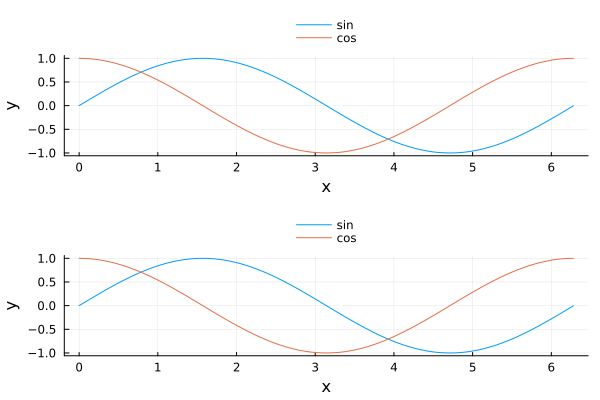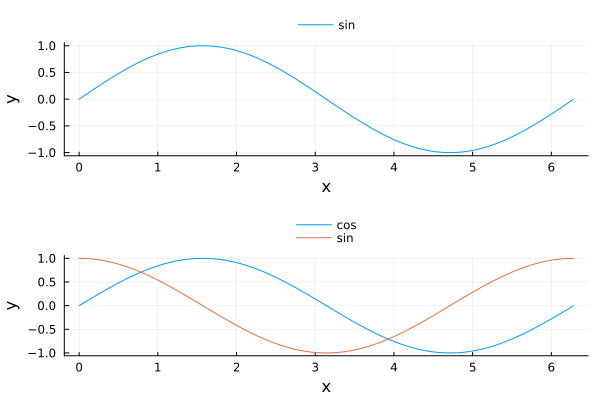# Line labels in subplot impacted by other subplots

I have a plot recipe that creates a few subplots. However, the labels that are displayed in the legend are not in the correct order in some cases and I do not understand what is wrong.

For example, in this MWE, the function plot_works() produces the correct result while plot_backward() has the sin and cos labels in subplot 2 reversed. The only difference in the recipes is that plot_works() plots both the sin and cos functions in subplot 1 and plot_backwards() only plots the sin function.

``````userplot Plot_Works
@recipe function f(h::Plot_Works)

# set up the subplots
layout --> (2,1)
foreground_color_legend --> nothing
legend --> :outertop

# Create data for plots
t = 0:0.01:2*pi
y1 = sin.(t)
y2 = cos.(t)

@series begin
subplot := 1
label := ["sin" "cos"]
xaxis := ("x")
yaxis := ("y")
x=t
y=[y1, y2]
x,y
end

@series begin
subplot := 2
label := ["sin" "cos"]  ##### Order reversed on plot
xaxis := ("x")
yaxis := ("y")
x=t
y=[y1, y2]
x,y
end
end

@userplot Plot_Backward
@recipe function f(h::Plot_Backward)

# set up the subplots
layout --> (2,1)
foreground_color_legend --> nothing
legend --> :outertop

# Create data for plots
t = 0:0.01:2*pi
y1 = sin.(t)
y2 = cos.(t)

@series begin
subplot := 1
label := "sin"
xaxis := ("x")
yaxis := ("y")
x=t
y=y1
x,y
end

@series begin
subplot := 2
label --> ["sin" "cos"]  ##### Order reversed on plot
xaxis := ("x")
yaxis := ("y")
x=t
y=[y1, y2]
x,y
end
end

# Make plots
plot_works()
savefig("plot_works.png")

plot_backward()
savefig("plot_backward.png")
``````

Output of `plot_works()` (note both figures have sin and cos in correct order)Output of `plot_backward()` (note legend labels are reversed)It looks like this open issue and this one.

A workaround is given at the end: to construct only one series per `@series` block.

1 Like